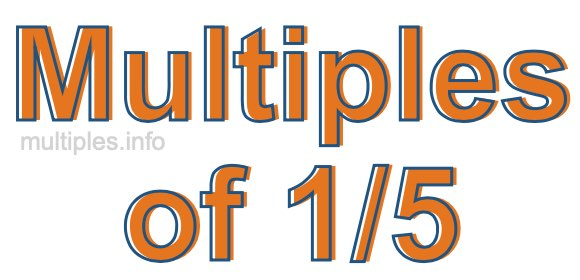Multiples of 1/5We define the multiples of 1/5 as the product of any integer multiplied by 1/5. Therefore, to create a list of multiples of 1/5 we start by multiplying 1/5 by one, then we multiply 1/5 by two, then we multiply 1/5 by three, and so on.

To multiply a fraction by an integer, we leave the denominator alone and multiply the numerator by the integer. It is impossible to give you a list of all multiples of 1/5 because the list goes on forever. However, here is the math to calculate the beginning list of multiples of 1/5.

1/5 × 1 = 1/5

1/5 × 2 = 2/5

1/5 × 4 = 3/5

1/5 × 4 = 4/5

1/5 × 5 = 5/5

1/5 × 6 = 6/5

1/5 × 7 = 7/5

1/5 × 8 = 8/5

1/5 × 9 = 9/5

1/5 × 10 = 10/5

1/5 × 11 = 11/5

1/5 × 12 = 12/5

We have simplified the fractions above for you. Below are the multiples of 1/5 in their lowest possible terms:

1/5 = 1/5

2/5 = 2/5

3/5 = 3/5

4/5 = 4/5

5/5 = 1

6/5 = 1 1/5

7/5 = 1 2/5

8/5 = 1 3/5

9/5 = 1 4/5

10/5 = 2

11/5 = 2 1/5

12/5 = 2 2/5

As we stated above, the list of multiples of 1/5 goes on forever because the product of 1/5 multiplied by any whole number (integer) is a multiple of 1/5. You can also create a list of multiples of 1/5 if you keep adding 1/5 like this:

1/5
1/5 + 1/5 = 2/5
1/5 + 1/5 + 1/5 = 3/5
1/5 + 1/5 + 1/5 + 1/5 = 4/5

Again, the list can go on forever, because you can continue adding 1/5 to the list. Therefore, the list of multiples of 1/5 is ∞ (infinite).

Multiples of Fractions
Do you need the multiples of another fraction? Enter a another fraction below to get the multiples of that fraction.

/

Multiples of 1/6
Go here for the next fraction on our list that we have multiples for.